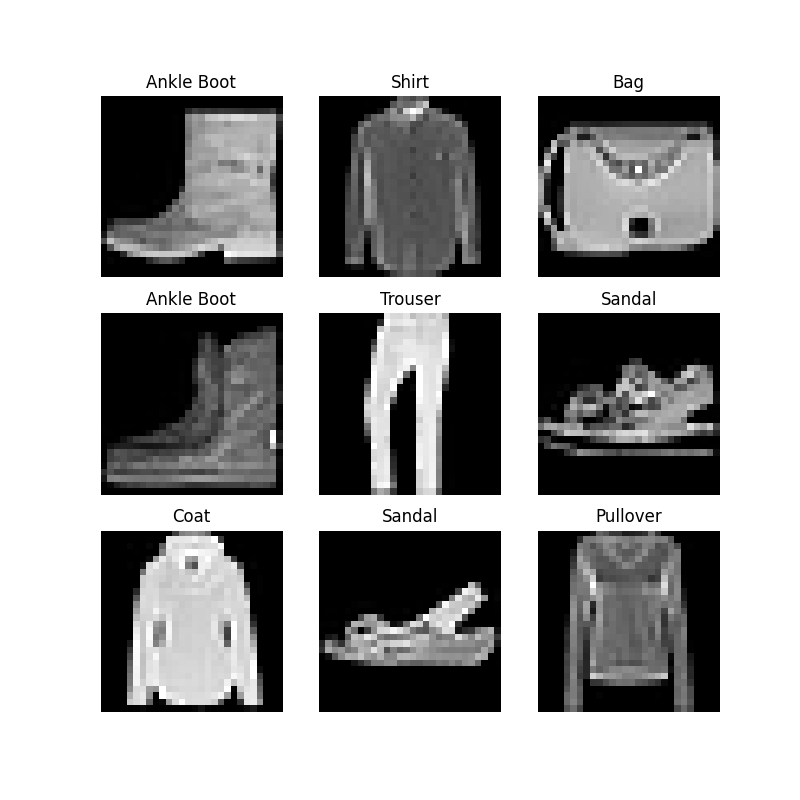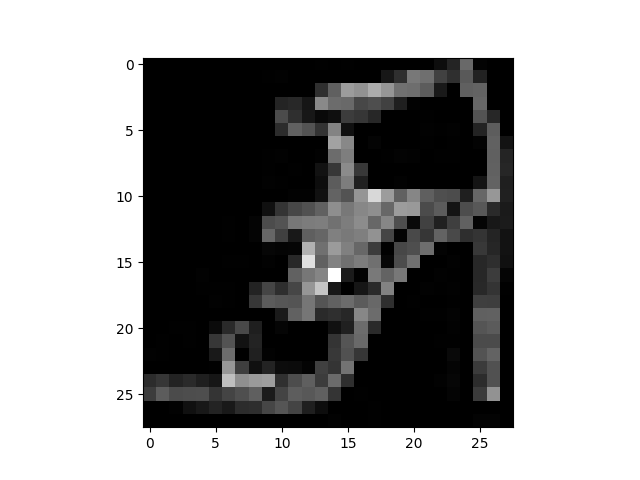# 数据集和数据加载器

PyTorch 领域库提供了一些预加载的数据集（如FashionMNIST），这些数据集是 torch.utils.data.Dataset 的子类，并实现特定数据的功能。它们可以被用来为你的模型制作原型和基准。你可以在这里找到它们：Image DatasetsText DatasetsAudio Datasets

## 加载一个数据集

• root 是存储训练/测试数据的路径,
• train 指定训练或测试数据集,
• download=True 如果 root 指定的目录没有数据，就自动从网上下载数据。
• transformtarget_transform 指定特征和标签的转换。
import torch
from torch.utils.data import Dataset
from torchvision import datasets
from torchvision.transforms import ToTensor
import matplotlib.pyplot as plt

training_data = datasets.FashionMNIST(
root="data",
train=True,
transform=ToTensor()
)

test_data = datasets.FashionMNIST(
root="data",
train=False,
transform=ToTensor()
)


## 迭代和可视化数据集

labels_map = {
0: "T-Shirt",
1: "Trouser",
2: "Pullover",
3: "Dress",
4: "Coat",
5: "Sandal",
6: "Shirt",
7: "Sneaker",
8: "Bag",
9: "Ankle Boot",
}
figure = plt.figure(figsize=(8, 8))
cols, rows = 3, 3
for i in range(1, cols * rows + 1):
sample_idx = torch.randint(len(training_data), size=(1,)).item()
img, label = training_data[sample_idx]
plt.title(labels_map[label])
plt.axis("off")
plt.imshow(img.squeeze(), cmap="gray")
plt.show()## 为你的文件创建一个自定义数据集

import os
import pandas as pd

class CustomImageDataset(Dataset):
def __init__(self, annotations_file, img_dir, transform=None, target_transform=None):
self.img_dir = img_dir
self.transform = transform
self.target_transform = target_transform

def __len__(self):
return len(self.img_labels)

def __getitem__(self, idx):
img_path = os.path.join(self.img_dir, self.img_labels.iloc[idx, 0])
label = self.img_labels.iloc[idx, 1]
if self.transform:
image = self.transform(image)
if self.target_transform:
label = self.target_transform(label)
return image, label


### init

tshirt1.jpg, 0
tshirt2.jpg, 0
......
ankleboot999.jpg, 9

def __init__(self, annotations_file, img_dir, transform=None, target_transform=None):
self.img_dir = img_dir
self.transform = transform
self.target_transform = target_transform


### len

Example:

def __len__(self):
return len(self.img_labels)


### getitem

def __getitem__(self, idx):
img_path = os.path.join(self.img_dir, self.img_labels.iloc[idx, 0])
label = self.img_labels.iloc[idx, 1]
if self.transform:
image = self.transform(image)
if self.target_transform:
label = self.target_transform(label)
return image, label


Dataset 每次加载一组我们数据集的特征和标签样本。在训练一个模型时，我们通常希望以 "小批量" 的方式传递样本，在每个训练周期重新打乱数据以减少模型的过拟合，并使用 Python 的 multiprocessing 来加快数据的加载速度。

DataLoader 是一个可迭代的对象，它用一个简单的API为我们抽象出这种复杂性。

from torch.utils.data import DataLoader



# 显示图像和标签。
print(f"Feature batch shape: {train_features.size()}")
print(f"Labels batch shape: {train_labels.size()}")
img = train_features.squeeze()
label = train_labels
plt.imshow(img, cmap="gray")
plt.show()
print(f"Label: {label}")Feature batch shape: torch.Size([64, 1, 28, 28])
Labels batch shape: torch.Size()
Label: 5